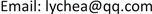—基于最大熵原理1. 引言

2. 熵与最大熵原理

S = k log W , (2.1)

H = − K ∑ i P i ln P i     . (2.2)

1) 离散型

H = − ∑ i = 1 n p i ln p i . (2.3)

∑ i = 1 n p i = 1 ,   p i ≥ 0 , i = 1 , 2 , ⋯ , n   . (2.4)

∑ i = 1 n p i = 1 , p i ≥ 0 , i = 1 , 2 , ⋯ , n   ; ∑ i = 1 n p i x i = E . (2.5)

2) 连续型

H = − ∫ f ( x ) ln f ( x ) d ( x ) . (2.6)

H = ∫ f ( x ) d ( x ) = 1 ,   ( f ( x ) ≥ 0 ) . (2.7)

∫ f ( x ) d x = 1   ; ∫ x f ( x ) d x = E . (2.8)

3. 心理距离与决策行为关系模型构建

W = ( P p 0 , p 1 , ⋯ , p n ) = P ! / ∏ i = 0 n p i ! . (3.1)

H = ln W = ln P !   − ∑ i = 0 n ln p i ! . (3.2)

∑ i = 0 n p i = P ; (3.3)

∑ i 2 π i p i = P 0 , (3.4)

L ( p i ) = ln P !   − ∑ i = 0 n ln p i ! + λ 1 ( P − ∑ i = 0 n p i ) + λ 2 ( P 0 − ∑ i = 0 n 2 π i p i ) . (3.5)

p i = e − λ 1 ⋅ e − 2 π i λ 2 . (3.6)

∫ 0 R 2 π r e − λ 1 ⋅ e − 2 π r λ 2 d r = P 0 . (3.7)

p ( r ) = 2 π λ 2 2 P 0 e − 2 π r λ 2 . (3.8)

ρ 0 = 2 π λ 2 2 P 0 (3.9)

ρ 0 = P 0 2 π r 0 2 . (3.10)

p ( r ) = ρ 0 e − r / r 0 . (3.11)

P D = α T D + β S s o c i a l D + γ S s p a t i a l D , (3.12)

p ( x , y , z ) = ρ 0 e − ( α x + β δ y + γ z ) / r 0 . (3.13)

p ′ ( x , y , z ) = ρ ′ 0 e − ( α ′ x + β ′ y + γ ′ z ) / r 0 . (3.15)

υ = p ′ ( x , y , z ) p ( x , y , z ) = ρ ′ 0 / ρ ⋅ e ( ( α − α ′ ) x + ( β − β ′ ) y + ( γ − γ ′ ) z ) / r 0 (3.16)

4. 模型验证

Measure of time distanc

5三个月以上
4三个月
3一个月
2一周
1一天

Measure of social distanc

7当有需要时，我首选该网络商家处浏览产品，不与其他网商对比，有喜欢的直接购买
6当有需要时，我愿意浏览该网络商家的产品
5当所选的产品与其他网络商家的标价一致，商品评价、细节图等其他因素也一致时，我愿意在该网商处购买
4当所选的产品高于其他网络商家等标价的0%~1%，但商品评价好于上述两者时，我愿意选择在该网络商家处购买
3当所选的产品高于其他网络商家等标价的1%~5%，但商品评价好于上述两者时，我愿意选择在京东商城购买
2当所选的产品高于其他网络商家等标价的5%，但商品评价好于上述两者时，我仍然愿意在该网络商家处购买
1当该网络商家的品牌形象、商品质量等出现信任危机后，我愿意继续在此购买产品

Measure of spatial distanc

85~7天
43~5天
21~3天
10~1天

Results of fitting curve in (3.18
Parameters1.0000−0.1786−0.1786−0.1785
Residuals (1.0e−04*)−0.0165−0.11240.1411−0.0165

5. 结论